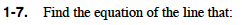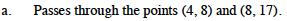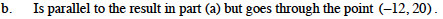### Home > PC > Chapter 1 > Lesson 1.1.1 > Problem1-7

1-7.What are two equation forms for a line?

y = mx + b where m = slope and b = y-intercept
(yy1) = m(xx1) where m = slope and (x1, y1) is a point.

How do you find slope from two points?

$\frac{\textit{y}_2-\textit{y}_1}{\textit{x}_2-\textit{x}_1}$What do you know about the slopes of two parallel lines?

Use one of the equation forms in part (a) above to find the equation.

They are the same.